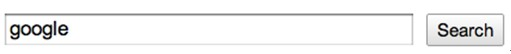# 如何更好地控制input输入框的高度

CSS 2 . 1 does not define which properties apply to form controls and frames, or how CSS can be used to style them. User agents may apply CSS properties to these elements. Authors are recommended to treat such support as experimental. A future level of CSS may specify this further.mac 下 webkit 的按钮不好控制浏览器 height + padding-top + padding-bottom + border-top-width + border-bottom-width IE6(xp) 21 + 2 + 1 + 2 + 2 = 28 IE7(xp) 21 + 2 + 1 + 2 + 2 = 28 IE8(win7) 21 + 2 + 1 + 2 + 2 = 28 Firefox 3.5(xp) 21 + 2 + 1 + 2 + 2 = 28 Firefox 3.5(win7) 23 + 2 + 1 + 1 + 1 = 28 Firefox 3.5(mac 10.6.2) 19 + 2 + 1 + 3 + 3 = 28 Firefox 3.5(ubuntu 10.04) 19 + 2 + 1 + 3 + 3 = 28 Chrome 5(xp) 21 + 2 + 1 + 2 + 2 = 28 Chrome 5(win7) 21 + 2 + 1 + 2 + 2 = 28 Chrome 5(mac 10.6.2) 21 + 2 + 1 + 2 + 2 = 28 Chrome 5(ubuntu 10.04) 21 + 2 + 1 + 2 + 2 = 28

 浏览器 height + padding-top + padding-bottom + border-top-width + border-bottom-width IE6(xp) 28 + 2 + 1 + 2 + 2 = 35 IE7(xp) 28 + 2 + 1 + 2 + 2 = 35 IE8(win7) 28 + 2 + 1 + 2 + 2 = 35 Firefox 3.5(xp) 28 + 2 + 1 + 2 + 2 = 35 Firefox 3.5(win7) 28 + 2 + 1 + 1 + 1 = 32 Firefox 3.5(mac 10.6.2) 28 + 2 + 1 + 3 + 3 = 37 Firefox 3.5(ubuntu 10.04) 28 + 2 + 1 + 3 + 3 = 37 Chrome 5(xp) 28 + 2 + 1 + 2 + 2 = 35 Chrome 5(win7) 28 + 2 + 1 + 2 + 2 = 35 Chrome 5(mac 10.6.2) 28 + 2 + 1 + 2 + 2 = 35 Chrome 5(ubuntu 10.04) 28 + 2 + 1 + 2 + 2 = 35

 浏览器 height + padding-top + padding-bottom + border-top-width + border-bottom-width IE6(xp) 18 + 3 + 3 + 2 + 2 = 28 IE7(xp) 18 + 3 + 3 + 2 + 2 = 28 IE8(win7) 18 + 3 + 3 + 2 + 2 = 28 Firefox 3.5(xp) 19 + 3 + 3 + 2 + 2 = 29 Firefox 3.5(win7) 19 + 3 + 3 + 1 + 1 = 27 Firefox 3.5(mac 10.6.2) 20 + 3 + 3 + 3 + 3 = 32 Firefox 3.5(ubuntu 10.04) 19 + 3 + 3 + 3 + 3 = 31 Chrome 5(xp) 19 + 3 + 3 + 2 + 2 = 29 Chrome 5(win7) 19 + 3 + 3 + 2 + 2 = 29 Chrome 5(mac 10.6.2) 18 + 3 + 3 + 2 + 2 = 28 Chrome 5(ubuntu 10.04) 19 + 3 + 3 + 2 + 2 = 29

 浏览器 height IE6(xp) 16 IE7(xp) 16 IE8(win7) 16 Firefox 3.5(xp) 16 Firefox 3.5(win7) 16 Firefox 3.5(mac 10.6.2) 16 Firefox 3.5(ubuntu 10.04) 16 Chrome 5(xp) 16 Chrome 5(win7) 16 Chrome 5(mac 10.6.2) 16 Chrome 5(ubuntu 10.04) 16

 Browser Lowest Version Support of Internet Explorer 8.0 box-sizing Firefox (Gecko) 1.0 (1.0) -moz-box-sizing Opera 7.0 box-sizing Safari (WebKit) 3.0 (522) -webkit-box-sizing